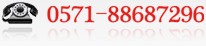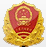﻿ Vertical injection molding machine molded article shrinkage (X)-Industry News-TAYU Injection Molding Machine Co.,Ltd.
Injection Molding Machine Mmanufacturers Tayu Machinery Product: Vertical Injection Molding Machine Bakelite Injection Molding Machine BMC injection molding machine# Your current location：home > Tayu News > Industry News Industry News

## Vertical injection molding machine molded article shrinkage (X)

Date：2015/10/27 0:07:27

Chapter V gating system and vertical injection molding machine injection mold cavity meshing
5.1 vertical injection molding machine gating system
5.1.1 sprue
Vertical injection molding machine injection mold sprue generally take a conical shape. Let the main channel of the nozzle in contact with the small end diameter d, main channel length tong, when the meshing of the sprue, the sprue axis direction divided into seg segment, and then along the radial direction of the main road shaft section half divided into m layer (for clarity, of the thickness direction dimension were significantly exaggerated) to form a two-dimensional array of grid nodes (seg, m).
5.1.2 shunt
Injection mold often to achieve change the flow direction of the flow area of ​​multi-level runner. Let's series runner for the grade. Here shunt include gate. For the first runner of the stage (j = 1tograde), if the cross-sectional dimensions vary, so this class runner is usually conical shape, whose cross section is circular, then the meshing of the runner and the sprue same; if it does not change the cross-sectional dimensions, then the runner cross-section is generally semi-circular, U-shaped, trapezoidal and rectangular, in cross-sectional area is obtained and this is equal to the radius of the circular cross-section r. Instead of cylindrical flow channel and along the axial direction thereof divided into sg segment number stored in each level of the longitudinal direction of the shunt was asked aliquot), and then along the radial direction of the cylindrical shaft section of the flow channel half divided into m layer, formed for the mesh node (i, h), number i is the basis of the previous level, the flow channel to continue ordering.
J-class runner with starting end of the spatial coordinates of the center of the spatial coordinates of the center point and the end of the insert d, a few (Sichuan to determine the spatial position of this class runner. If the starting end of the first j-class runner and the upper-level flow the end of the road in the spatial positions coincide, and a cross-sectional radius are equal, then the flow direction of the j-level runner and runner on the same level, the size of flow cross-section of the transition occurs changes as shown in Figure 5, if not a case of 2 , then there are two possibilities: one case the flow direction of the j-class runner and the runner on the same level, but the size of the cross section of the flow of a sudden change; the flow direction of another case j-level runner with respect to changes occur on primary flow path. In both cases, you need at j level shunt flow path between the starting end of the end of the level and add a transition section, is about the relationship between the actual flow path into the direction of flow constant , case flow cross-section of a smooth transition, so that you can program the plastic melt flow within the gating system as a one-dimensional flow to deal with. to the transition period, the first runner mesh nodes j stage in the flow direction of the number i value is incremented by one.
After pouring system to be set analysis region meshing array of nodes reached (roral, m).

5.2 injection mold cavity plastic melt flow front Determination
The second chapter analyzes based on shrinkage of injection molded products vertical injection molding machine to the conclusion, the points of injection molded articles is shrunk along the flow path, that is a point in the flow path contraction path, determining the flow of each point path is to analyze the injection molding process shrinkage premise. Theoretically, cutting the flow path of a point to point on behalf of the fluid particle velocity direction, to cut the flow path and tangential melt front should be orthogonal, in the specific division of the flow path, although not completely do it to determine the melt front location still has great significance for the division of the flow path. First discussions single gate. If the gate reaches the same time melt injection points required for a cavity in the flow field, then these two points will be located on the melt front the same time. Fundamental factors determine the flow of time is the flow distance and flow rate, the analysis begins with the melt front position both aspects.
Gate located O points, A, B are two points which flows within the region, from the gate to reach the A, B, respectively, the shortest distance is equal to the flow line OA, the length of the mouth ugly. If injectors = ugly mouth, then make the plastic melt flow path and a path opening knife OA time differences factors are: the cavity thickness of these two paths, mold wall temperature and the degree of change in the direction of flow. The thickness of the cavity that path larger flow resistance is small, so the larger flow, but also the larger the volume to be filled, so the leading edge of its flow front is not very obvious; larger cavities where mold wall thickness temperature will be higher, farther away from the cooling circuit mold wall temperature will be higher, mold wall temperature is high, the slow cooling of the melt in the cavity, a small viscosity, the flow resistance is reduced, but in a short mold filling process wall temperature difference is not obvious, so ignore the flow resistance of the resulting differences in their Excluding; changing the flow direction of a significant increase in the flow resistance, the flow rate is reduced, which affect the flow of time equivalent to the flow distance is extended, it can flow degree change of direction to get the equivalent distance on the shortest distance corrected flow impact to characterize the flow direction of the change on the flow of time.
After calculating the mold cavity surface of each pitch distance gate equivalent, the equivalent value of the connection from the same point up, as the melt front position of each time step. If there is a core presence, and the gate is located opposite side of the core point will be two ways to calculate the equivalent distance to arrive at a distance of two equivalent value, if they are different, whichever is less, as the equivalent distance of this point ; if they are the same, indicating that this point is located on the weld marks. When there are multiple gates, each gate if the gating system relative to the upper level and the same uniform cross-sectional area, you can still calculate the equivalent distance of each pitch different gate as described above, and two adjacent gates point is equal to the equivalent distance even as weld marks between the two gates in order to determine the flow area of ​​each gate; if each gate runner system with respect to the previous uniform cross-sectional area but different, in comparison a point of time when the plastic melt in the mold cavity surface needed to reach via different gates, but also to the gate of the cross-sectional area taken into account, the degree of change in accordance with a dot pitch of the j-th gate shortest distance flow and flow direction The calculated equivalent weight multiplied by the distance (Sav. / sj), as a new value of this pitch distance equivalent j-th gate, Save represents the arithmetic mean of the cross sectional area of ​​each gate, on behalf of the j-th gate winter The cross-sectional area; if each gate with respect to the non-uniform distribution of primary gating system, the press no swelling effect calculated with respect to the j-th gate gating system equivalent to the previous distance, then put it with the cavity a point relative to the first gate a distance equivalent, as this point via the j-th gate with respect to the distance value equivalent level gating system, and by this value judgment melt front position and weld line location. In the pouring system meshing module liudao () to add each point on the center line of the flow channel relative to the main road entrance to the equivalent distance le (i) (i two 0tototal) calculations, and le (i) stored in the data file jiaole .txt.

Return